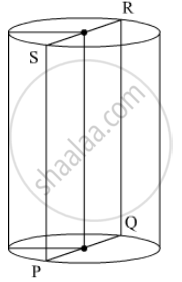# Vertical Cross-section of a Right Circular Cylinder is Always a - Mathematics

MCQ

Vertical cross-section of a right circular cylinder is always a

• square

• rectangle

• rhombus

• trapezium

#### Solution

From the following figure of cylinder we observe that the vertical cross section is the rectangle PQRS.Concept: Surface Area of Cylinder
Is there an error in this question or solution?

#### APPEARS IN

RD Sharma Mathematics for Class 9
Chapter 19 Surface Areas and Volume of a Circular Cylinder
Exercise 19.4 | Q 4 | Page 28

Share